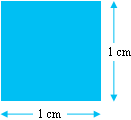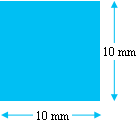SEARCH HOMEMath Central Quandaries & QueriesQuestion from David, a parent: How do you convert square mm to square cm?One square centimeter is literally a square with side lengths of 1 centimeter:One centimeter is equal to 10 millimeters, therefore this square also has a length of 10 mm on each side:If you divided each side into millimeter long segments, you would see that one square centimeter is equal to 100 square millimeters (100 squares with side lengths of 1 mm).  Or simply,

$1 cm^2 = 1 cm \times 1 cm = 10 mm \times 10 mm = 100 mm^2$

To convert from square millimeters to square centimeters, you simply divide by 100.  For example,

$1670 mm^2 = 16.70 cm^2 .$

TylerMath Central is supported by the University of Regina and the Imperial Oil Foundation.